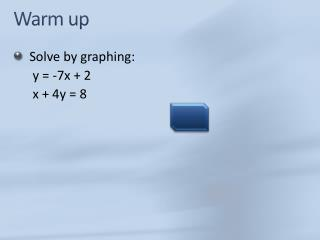DownloadDownload PresentationWarm up

# Warm up

Download Presentation## Warm up

- - - - - - - - - - - - - - - - - - - - - - - - - - - E N D - - - - - - - - - - - - - - - - - - - - - - - - - - -
##### Presentation Transcript

1. Warm up • Solve by graphing: y = -7x + 2 x + 4y = 8 (0,2)

2. Lesson 9-2 The Substitution Method Objective: To use the substitution method to solve systems of linear equations.

3. Solving a system of equations by substitution Pick the easier equation. The goal is to get y= ; x= ; etc. Step 1: Solve an equation for one variable. Put the equation solved in Step 1 into the other equation. Step 2: Substitute Get the variable by itself. Step 3: Solve the equation. Substitute the value of the variable into the equation. Step 4: Plug back in to find the other variable. Step 5: Check your solution. Substitute your ordered pair into BOTH equations.

4. Solve the system using substitution x + y = 5 y = 3 + x Step 1: Solve an equation for one variable. The second equation is already solved for y! Step 2: Substitute x + y = 5x + (3 + x) = 5 2x + 3 = 5 2x = 2 x = 1 Step 3: Solve the equation.

5. Solve the system using substitution x + y = 5 y = 3 + x Step 4: Plug back in to find the other variable. x + y = 5 (1) + y = 5 y = 4 (1, 4) (1) + (4) = 5 (4) = 3 + (1) Step 5: Check your solution. The solution is (1, 4). What do you think the answer would be if you graphed the two equations?

6. 3) Solve the system using substitution x = 3 – y x + y = 7 Step 1: Solve an equation for one variable. The first equation is already solved for x! Step 2: Substitute x + y = 7 (3 – y) + y = 7 3 = 7 The variables were eliminated!! This is a special case. Does 3 = 7? FALSE! Step 3: Solve the equation. When the result is FALSE, the answer is NO SOLUTIONS.

7. 3) Solve the system using substitution 2x + y = 4 4x + 2y = 8 The first equation is easiest to solved for y! y = -2x + 4 Step 1: Solve an equation for one variable. Step 2: Substitute 4x + 2y = 8 4x + 2(-2x + 4) = 8 4x – 4x + 8 = 8 8 = 8 This is also a special case. Does 8 = 8? TRUE! Step 3: Solve the equation. When the result is TRUE, the answer is INFINITELY MANY SOLUTIONS.

8. Practice • 2x + y = 9 x + 4y = 1 • x – 2y = y • 2x – 5y = 4 • y = 4x -5 • 8x – 2y = 20 (5, -1) (12, 4) (No soln)

9. Word Problems • The sum of two numbers is 5. The larger number exceeds twice the smaller number by 14. Find the numbers.

10. Word Problems • A pet shop sold 23 puppies and kittens one week. They sold 9 more puppies than kittens. How many of each did they sell?

11. Word Problems • Jeannette has 20 nickels and dimes worth \$1.75. How many of each coin does she have?